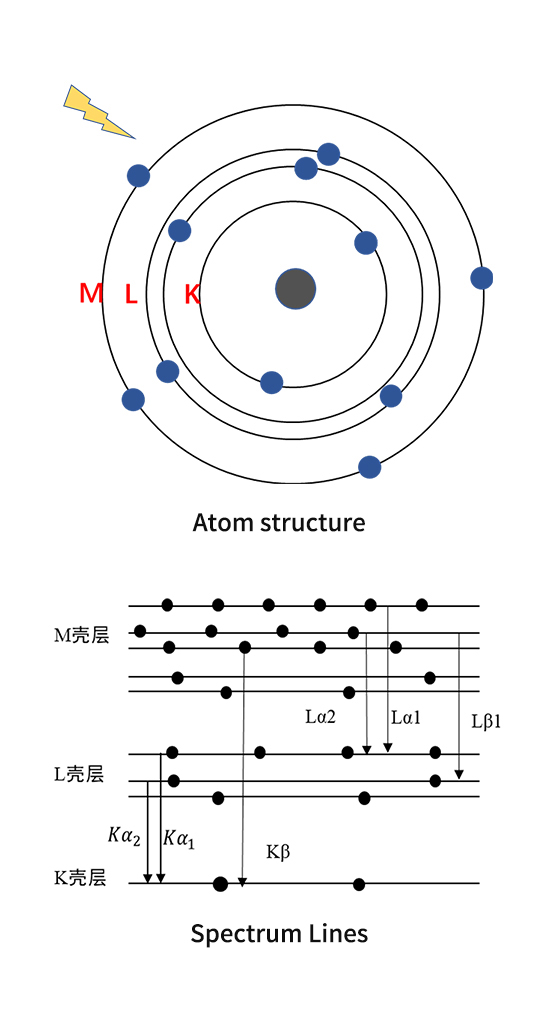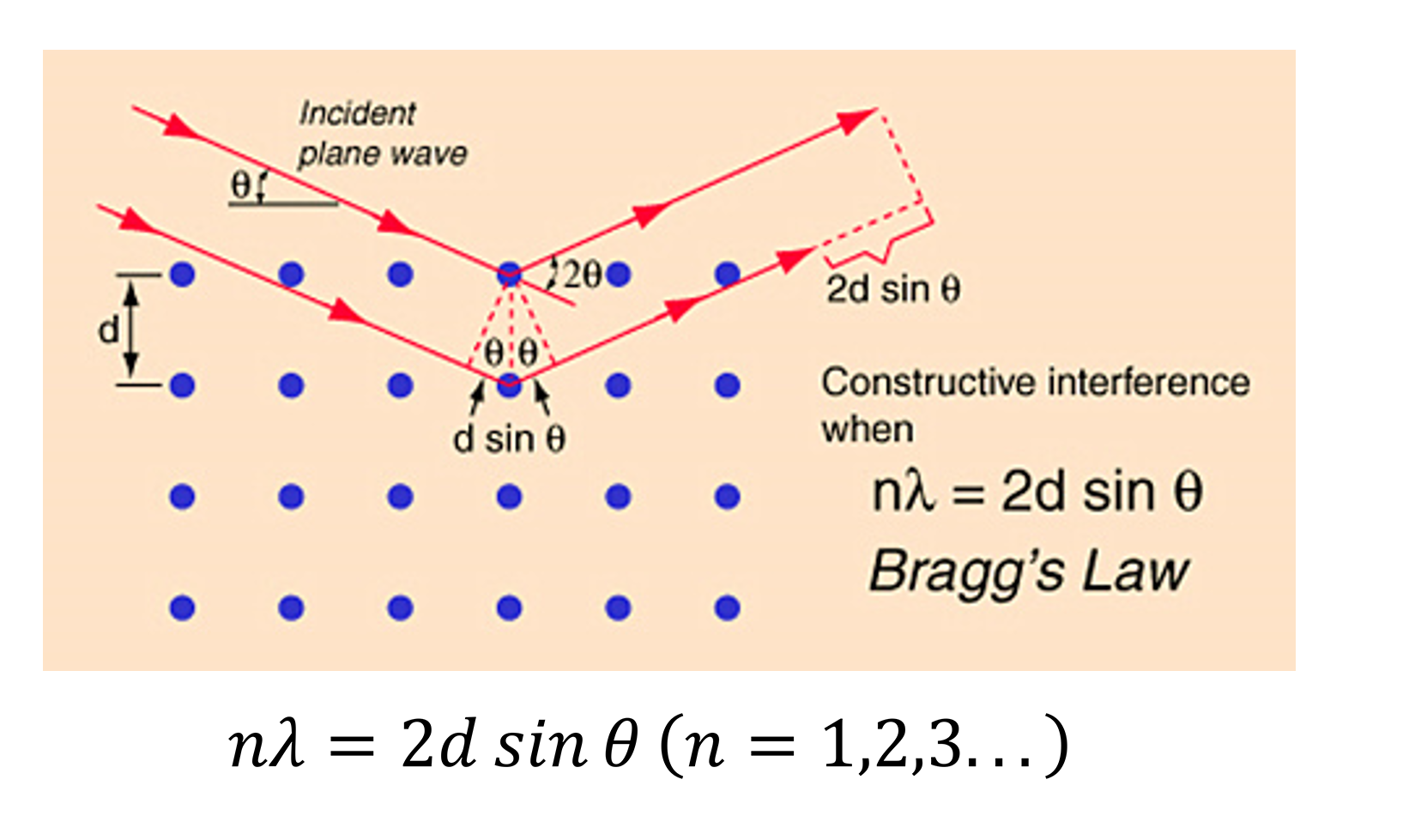﻿ Principle of X-ray fluorescence spectrometry - XRF KNOWLEDGE - Beijing Ancoren Technology Co.,LTD
Beijing Ancoren Technology Co.,LTD
• 中文
• English
•

### XRF LIBRARY

##### Location：Home > XRF > XRF KNOWLEDGE
Principle of X-ray fluorescence spectrometry
2017-06-21 20:22:201、X-ray fluorescence

X-ray fluorescence is the X-ray emitted by an object under X-ray irradiation, which contains information of the chemical composition. The instrument that determines the chemical compositions of the items by analyzing the X-ray fluorescence is the X-ray fluorescence analyzer.
From the perspective of atomic physics, each chemical element has its specific atomic energy level structure. When a beam of high-energy particles hit against an atom and the energy is greater than or equal to the binding energy of a certain orbital electron of the atom, the orbital electron can be expelled to form a hole, leaving the atom in an excited state, because of the unstable state, the outer electrons transition to holes will carry out suddenly, that is called the relaxation process, the relaxation process can be either a non-radiation transition or a radiation transition, the former process in which the electron with energy in the X-ray range undergoes a transition in which a second electron is emitted rather than an X-ray photon is the Auger effect; and the latter in which the energy is not absorbed by the other atoms, but emitted in the form of radiation is X-ray fluorescence. When the holes generate in K layers, the electrons in different outer layers (L, M, N ... layers) traverse to the holes, corresponding to K-lines, Similarly, holes in the L layer, L-lines are formed.
The energy of the X-ray fluorescence is irrelevant with the incident energy, only depends on the difference between the two energy levels which is completely determined by the essential structure of the atom, so it is the characteristic X-ray, the energy we know and the element we find out.

As we all know the characteristic X-ray energy is directly determined by the difference in atomic orbital energy levels, according to the Quantity Energy equation:Where E refers to the photon energy, n is the frequency, and h is the Planck constant.
Hence one can writeAccording to Moseley's law, the frequency is proportional to the second power of the atomic numberTherefore, knowing the energy or wavelength of the characteristic X-ray, you can identify what the element is. This is the most basic principle of X-ray fluorescence spectroscopy (wavelength dispersion and energy dispersion spectrum analyzer).

2、Fundamental theories of X-ray fluorescence spectroscopy

1)   Moseley's law
Moseley's Law is an experimental law reflecting the principle of the characteristic X-ray spectrum of each element. In 1913, Moseley studied spectrum K and L lines of 38 elements from Al to Au, discovering that Frequency of the characteristic X-ray for elements is directly proportional to the square of the atomic number, Z, which shows they are in one-to-one correspondence, making X-ray fluorescence analysis technology one of the most reliable methods for qualitative analysis.2)   Bragg's Law
Bragg's Law is a law reflecting the basic relationship of crystal diffraction. This is also the working principle of a wavelength-type X-ray fluorescence spectroscopic crystal, so wavelengths of the characteristic X fluorescence of different elements are separated to achieve qualitative analysis.3)
Berr-Lambert’s Law
Berr-Lambert ’s Law is a law that reflects the absorption of a sample, which involves the calculation of the theoretical relative intensity of X-ray fluorescence.

When X-rays pass through a object, due to the Photoelectric effect, Compton and Thermal effects, the intensity of X-ray will be attenuated, showing a change in energy and direction of movement, thereby reducing the number of X-ray photons along the direction incidence. This is called absorption.

Normally, the mass absorption coefficient has a mutation with the change of wavelength, and the wavelength at which the mutation occurs is called the absorption limit whicht increases with wavelength increase. And the matrix absorption enhancement effect plays a very important role in the quantitative analysis process.where I0  is Initial intensity of incident light, I is the intensity of emission, μ is Linear absorption coefficient, μm is Mass absorption coefficient

3、Theory of X-ray fluorescence quantitative analysis

The basis of quantitative analysis by X-ray fluorescence spectroscopy is that the fluorescence X-ray intensity Ii is proportional to the content Wi of the element in the sampleWhere Is is the intensity of the X-ray fluorescent of element i when Wi = 100%. According to the formula above, standard curve method, incremental method, internal standard method, etc. can be used for the quantitative analysis. However, the existence of absorption enhancement effect often makes the relationship between content and the net intensity of the fluorescence present a very complicated nonlinear relationship, In order to solve this problem, there are two main methods, one is the empirical coefficient method, and the other is the Fundamental parameter method.

Empirical coefficient method is a common method in X-ray fluorescence analysis. Which requests the standard samples should be enough and the range of the content covers the range of variation of the actual samples.

In Fundamental Parameter method all links from the occurrence to the detection of the spectrums will be calculated, which includes the X-ray tube's emission spectrum (target characteristic spectrum and continuous spectrum). incident spectrum after the filter Or monochromator entering into the sample, various matrix effects (matrix absorption effects, secondary fluorescence of coexisting elements), scattering effects (Rayleigh scattering and Compton scattering) on the sample, as well as effects (efficiency, escape peaks (fluorescence escape and Compton scattering escape), pulse accumulation, etc.) that occurred after the detector received X-rays emitted from the sample. In brief, the spectrum of the FP calculated is consistent with the actual spectrum measured with the detector.The Fundamental parameter method solves the nonlinear problems problem through software programming, and the matrix effect and the element absorption enhancement effect are fully calculated to obtain the correct quantitative result. The application of the basic parameter method greatly expands the application range, solving the problem of the standards less in some fields.